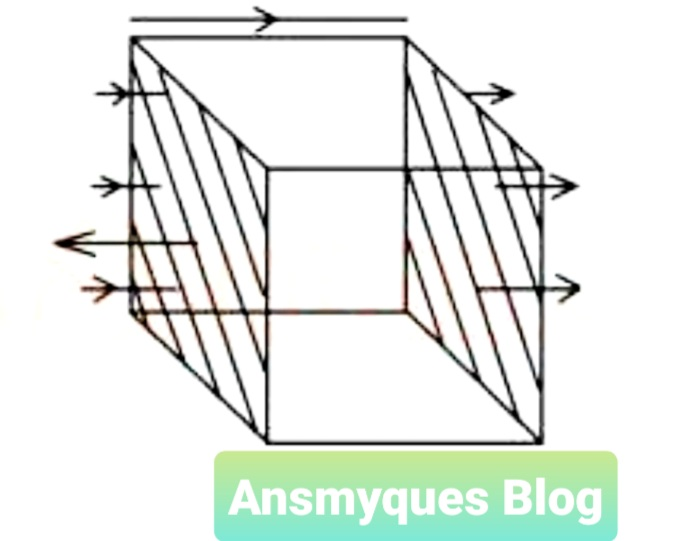# PHYSICS QUESTIONS AND ANSWERS (9)

1. The force on a current carrying conductor in a magnetic field is greatest when the

A. conductor makes an angle of 60° with the field

B. force is independent of the angles between the fields and the conductor

C. conductor is parallel with the field

D. conductor is at right angle with the field

2. Energy losses through eddy currents are reduced by using

A. low resistance wires

B. insulated soft iron wires

C. few turns of wires

D. high resistance wires

3. The magnetic force on a charged particle moving with velocity v is

A. proportional to both the magnitude of the charge and the velocity v

B. independent of the magnitude of the charge

C. proportional to the velocity v only

D. proportional to the magnitude of the charge only4. The diagram above shows a closed side 0.5m in a uniform electric field E in the direction shown by the arrows. What is the flux Φ for the box?

A. 0.5 E

B. 2.0 E

C. 0.2 E

D. 0.0 E

5. Which of the following ammeters may be used to measure alternating currents?​​

i. moving-coil ammeter​​

ii. moving-iron ammeter​​

iii. hot-wire ammeter

A. i and ii only

B. ii and iii only

C. i and iii only

D. i, ii and iii114

6. A circuit has an area of 0.4m2 and consists of 50 loops of wire. If the loops are twisted and allowed to rotate at a constant angular velocity of 10 rad s-1 in a uniform magnetic field of 0.4T, the amplitude of the induced voltage is

A. 8V

B. 16V

C. 20V

D. 80V

227

7. The force on a charge moving with velocity v in a magnetic field B is half of the maximum force when the angle between v and B is

A. 90o

B. 45o

C. 30o

D. 0o

8. The energy stored in an inductor of inductance 5mH when a current of 6A flows through it is

A. 9.0 x 10-3J

B. 9.0 x 10-2J

C. 1.4 x 10-2J

D. 1.8 x 10-3J

9. a proton moving with a speed of 1.0 x 106ms-1 through a magnetic field of 1.0T experiences a magnetic force of magnitude 8.0 x 10-14 N. The angle between the proton's velocity and the field is

A. 60° ​​ ​​ ​​ ​​ ​​ ​​ ​​ ​​ ​​ ​​​​ B. 90°

C. 30° ​​ ​​ ​​ ​​ ​​ ​​ ​​ ​​ ​​ ​​​​ D. 45°

10. Two inductors of inductances 5mH and 15mH are connected in series and a current of 5A flows through them. the total energy stored in the inductor is

A. 500.0J

B. 250.0J

C. 62.5J

D. 50.0J

E. no correct option

11. Which of the following is a property of steel?

A. it cannot retain its magnetism longer than iron

B. it can be used for making temporary magnets

C. it can be used for making permanent magnets

D. it can easily be magnetized and demagnetized

544

12. In measuring high frequency a.c, the instrument used is the

A. d.c ammeter

B. moving coil ammeter

C. moving iron ammeter

D. hot wire ammeter

13. What is the best method of demagnetizing a steel bar magnet?

A. Hammering

B. Heating it

C. Rough handling it

See also  Shugaba v Minister of Internal Affairs : Short summary and court held

D. Solenoid method

632

14. The magnitude of the angle of dip at the equator is

A. 360o

B. 0o

C. 90o

D. 180o

15. Induced emfs are best explained using

A. Ohm's law

C. Coulomb’s law

D. Lenz's law

16. Which of the following is required to convert a milliammeter to an ammeter?

A. A high resistance in parallel

B. A high resistance in series

C. A low resistance in parallel

D. A low resistance in series

844

17. The primary winding of a transformer has 400 turns and its secondary has 100 turns. If a source of e.m.f. of 12V is applied to the primary, the secondary e.m.f. will be

A. 3V

B. 6V

C. 24V

D. 48V

18. The angle between the direction of the earth's magnetic field and the horizontal is called the

A. angle of deviation

B. magnetic declination

C. magnetic meridian

D. angle of dip

950

19. A dynamo primarily combines

A. mechanical energy into electrical energy

B. electrical energy into kinetic energy

C. potential energy into kinetic energy

D. kinetic energy into potential energy

20. Which of the following statements is CORRECT about the earth's magnetic field?

A. the angle of dip is the angle which a freely suspended magnet makes with the vertical

B. the angle of declination is the angle between the magnetic meridian and the geographic meridian

C. the angle of declination is the angle which a magnetic compass makes with the magnetic meridian

D. the angle of inclination is the difference between the angle of dip and the angle of declination